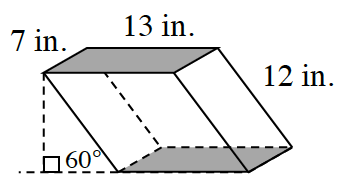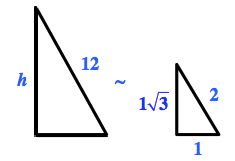### Home > CCG > Chapter 9 > Lesson 9.1.5 > Problem9-57

9-57.Elliot has a modern fish tank that is in the shape of an oblique prism, shown at right.

1. If the slant of the prism makes a $60°$ angle with the flat surface on which the prism is placed, find the volume of water the tank can hold. Assume that each base is a rectangle

Use a special right triangle to find the height of the parallelogram.Find the height of the parallelogram:

$\text{linear scale factor}=6$

$h=6(1\sqrt{3})=6\sqrt{3}$

Find the volume:

$\text{V} = 7 \cdot 13 \cdot 6\sqrt{3} = 546\sqrt{3}$

$\approx 945.7\: \text{in.}^3$

2. If Elliot has $25$ fish, how crowded are the fish? That is, what is the density of fish, measured in number of fish per cubic inch?

3. What is the density of fish in Elliot’s tank in fish per cubic foot?